# Tunable Bandpass IIR Filter

Design bandpass IIR filter with tunable filter specifications

Since R2023a

•Libraries:
DSP System Toolbox / Filtering / Filter Sources

## Description

Use the Tunable Bandpass IIR Filter block to design a bandpass IIR filter using the Butterworth, Chebyshev Type I and Chebyshev Type II design methods. You can tune the filter design specifications such as the filter order, 3-dB cutoff frequencies, passband ripple, and stopband attenuation during simulation. When the values of these parameters change, the block redesigns the filter and outputs the numerator and denominator coefficients in the second-order section (SOS) form (since R2023b) or the fourth-order section (FOS) form.

## Examples

expand all

Filter a noisy sinusoidal signal using the Fourth-Order Section Filter block. Obtain the numerator and denominator coefficients of the FOS filter using the Tunable Bandpass IIR Filter block.

Tune the frequency specifications of the FOS filter during simulation.

Open and Run Model

Open the fourthordersectionfilter.slx model by clicking the Open Model button.

The input signal in the model is a sum of two sine waves with the frequencies of 100 Hz and 350 Hz. The sample rate is 1000 Hz and the number of samples in each frame is 1024. The Random Source block adds zero-mean white Gaussian noise with a variance of 1e-4 to the sum of the sine waves.

The Tunable Bandpass IIR Filter block designs a sixth-order bandpass IIR filter with the first and second 3-dB cutoff frequencies of 0.25rad/sample and 0.75rad/sample, respectively. The block generates coefficients as a cascade of fourth-order sections. Visualize the frequency response of the filter using the Filter Visualizer.

Run the model.

The Fourth-Order Section Filter block filters the noisy sinusoidal signal. Visualize the original sinusoidal signal and the filtered signal using the Spectrum Analyzer.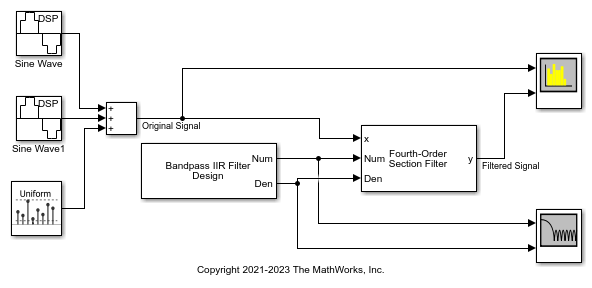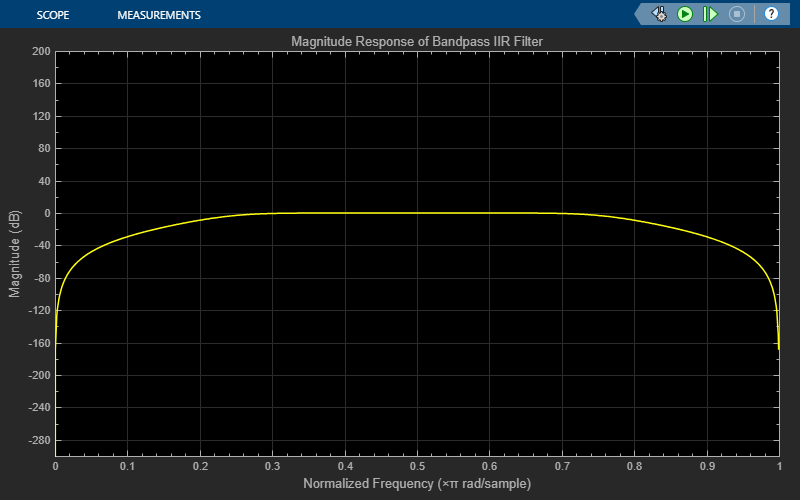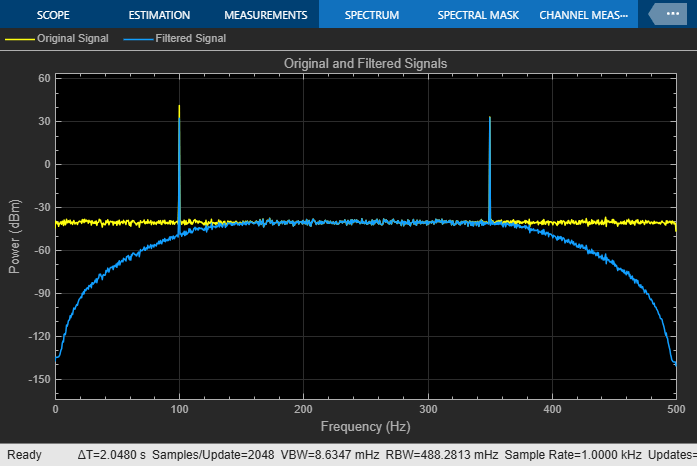Tune Frequency Specification of FOS Filter

During simulation, you can tune the frequency specifications of the FOS filter by tuning the frequency parameters in the Tunable Bandpass IIR Filter block. The frequency response of the FOS filter updates accordingly.

Change the first 3-dB cutoff frequency to 0.65rad/sample in the Tunable Bandpass IIR Filter block. The first tone of the sinusoidal signal is attenuated as it no longer falls in the passband region of the filter.Filter a noisy sinusoidal signal using the Second-Order Section Filter block. Obtain the numerator and denominator coefficients of the SOS filter using the Tunable Bandpass IIR Filter block.

Tune the frequency specifications of the SOS filter during simulation.

Open and Run Model

Open the secondordersectionfilter model by clicking the Open Model button.

The input signal in the model is a sum of two sine waves with the frequencies of 100 Hz and 350 Hz. The sample rate is 1000 Hz and the number of samples in each frame is 1024. The Random Source block adds zero-mean white Gaussian noise with a variance of 1e-4 to the sum of the sine waves.

The Tunable Bandpass IIR Filter block designs a sixth-order bandpass IIR filter with the first and second 3-dB cutoff frequencies of 0.25rad/sample and 0.75rad/sample, respectively. The block generates coefficients as a cascade of second-order sections. Visualize the frequency response of the filter using the Filter Visualizer.

Run the model.

The Second-Order Section Filter block filters the noisy sinusoidal signal. Visualize the original sinusoidal signal and the filtered signal using the Spectrum Analyzer.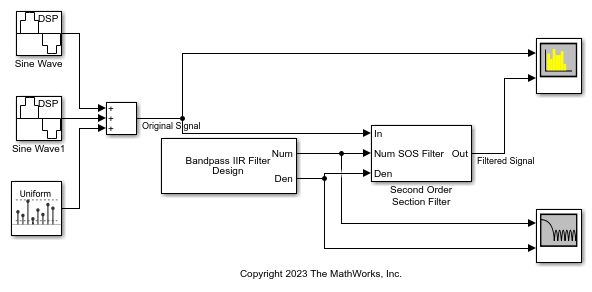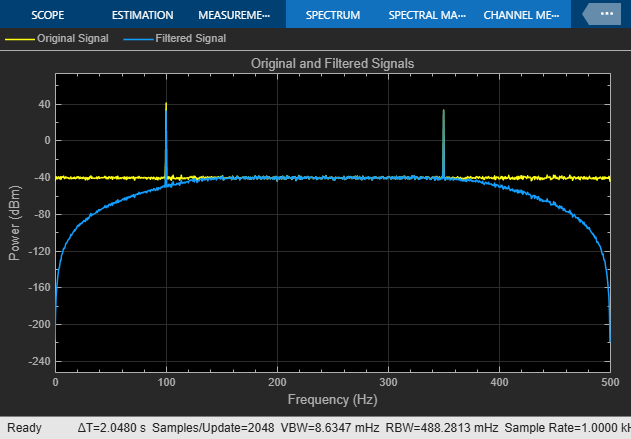Tune Frequency Specifications of SOS Filter

During simulation, you can tune the frequency specifications of the SOS filter by tuning the frequency parameters in the Tunable Bandpass IIR Filter block. The frequency response of the SOS filter updates accordingly.

Change the second 3-dB cutoff frequency to 0.4rad/sample in the Tunable Bandpass IIR Filter block. The second tone of the sinusoidal signal gets attenuated as it no longer falls in the passband region of the filter.## Ports

### Input

expand all

Specify the filter order as an even positive integer. You can change the filter order you input through this port during simulation.

#### Dependencies

To enable this port, select the Specify filter order from input port parameter.

Data Types: single | double | int8 | int16 | int32 | int64 | uint8 | uint16 | uint32 | uint64

Specify the first 3-dB cutoff frequency Fc1 of the filter in normalized frequency units as a nonnegative scalar less than or equal to the second 3-dB cutoff frequency Fc2.

You can change the first 3-dB cut off frequency you input through this port during simulation.

#### Dependencies

To enable this port, select the Specify first 3-dB cutoff frequency from input port parameter.

Data Types: single | double

Specify the second 3-dB cutoff frequency of the filter in normalized frequency units as a positive scalar greater than or equal to Fc1 and less than or equal to 1.

You can change the second 3-dB cut off frequency you input through this port during simulation.

#### Dependencies

To enable this port, select the Specify second 3-dB cutoff frequency from input port parameter.

Data Types: single | double

Specify the passband ripple of the Chebyshev Type I filter as a nonnegative scalar in dB. You can change the passband ripple you input through this port during simulation.

#### Dependencies

To enable this port:

• Set the Design method parameter to Chebyshev Type I.

• Select the Specify the passband ripple from input port parameter.

Data Types: single | double

Specify the stopband attenuation of the Chebyshev Type II filter as a nonnegative scalar in dB. You can change the stopband attenuation you input through this port during simulation.

#### Dependencies

To enable this port:

• Set the Design method parameter to Chebyshev Type II.

• Select the Specify the stopband attenuation from input port parameter.

Data Types: single | double

### Output

expand all

Numerator coefficients, returned as a P-by-3 matrix (SOS form) or a P-by-5 matrix (FOS form), where P is the maximum number of filter sections.

SOS form (since R2023b)

When you set Filter cascade sections form to Second-order sections, the block generates a P-by-3 numerator coefficients matrix.

$b=\left[\begin{array}{ccc}{b}_{01}& {b}_{11}& {b}_{21}\\ {b}_{02}& {b}_{12}& {b}_{22}\\ ⋮& ⋮& ⋮\\ {b}_{0P}& {b}_{1P}& {b}_{2P}\end{array}\right]$

This equation represents the SOS filter in the transfer function form.

$H\left(z\right)=\prod _{k=1}^{P}{H}_{k}\left(z\right)=\prod _{k=1}^{P}\frac{{b}_{0k}+{b}_{1k}{z}^{-1}+{b}_{2k}{z}^{-2}}{{a}_{0k}+{a}_{1k}{z}^{-1}+{a}_{2k}{z}^{-2}},$

where

• b is a matrix of numerator coefficients.

• a is a matrix of denominator coefficients that the block outputs at the Den output port.

• k is the row index.

P equals ceil(Nmax/2) in the SOS form, where Nmax is the value of the Filter maximum order (must be even) parameter.

When the actual filter order N is less than the maximum filter order Nmax, the last ceil(Nmax/2) − ceil(N/2) sections are trivial with coefficients [b0, b1, b2] = [1, 0, 0].

FOS form

When you set Filter cascade sections form to Fourth-order sections, the block generates a P-by-5 numerator coefficients matrix.

$b=\left[\begin{array}{ccccc}{b}_{01}& {b}_{11}& {b}_{21}& {b}_{31}& {b}_{41}\\ {b}_{02}& {b}_{12}& {b}_{22}& {b}_{32}& {b}_{42}\\ ⋮& ⋮& ⋮& \ddots & ⋮\\ {b}_{0P}& {b}_{1P}& {b}_{2P}& {b}_{3P}& {b}_{4P}\end{array}\right]$

This equation represents the FOS filter in the transfer function form.

$H\left(z\right)=\prod _{k=1}^{P}{H}_{k}\left(z\right)=\prod _{k=1}^{P}\frac{{b}_{0k}+{b}_{1k}{z}^{-1}+{b}_{2k}{z}^{-2}+{b}_{3k}{z}^{-3}+{b}_{4k}{z}^{-4}}{{a}_{0k}+{a}_{1k}{z}^{-1}+{a}_{2k}{z}^{-2}+{a}_{3k}{z}^{-3}+{a}_{4k}{z}^{-4}}$

P equals ceil(Nmax/4) in the FOS form.

When the actual filter order N is less than the maximum filter order Nmax, the last ceil(Nmax/4) − ceil(N/4) sections are trivial with coefficients [b0, b1, b2, b3, b4] = [1, 0, 0, 0, 0].

The data type of this port depends on the value of the Output data type parameter.

Data Types: single | double

Denominator coefficients, returned as a P-by-3 matrix (SOS form) or a P-by-5 matrix (FOS form), where P is the maximum number of filter sections.

SOS form (since R2023b)

When you set Filter cascade sections form to Second-order sections, the block generates a P-by-3 denominator coefficients matrix.

$a=\left[\begin{array}{ccc}{a}_{01}& {a}_{11}& {a}_{21}\\ {a}_{02}& {a}_{12}& {a}_{22}\\ ⋮& ⋮& ⋮\\ {a}_{0P}& {a}_{1P}& {a}_{2P}\end{array}\right]$

This equation represents the SOS filter in the transfer function form.

$H\left(z\right)=\prod _{k=1}^{P}{H}_{k}\left(z\right)=\prod _{k=1}^{P}\frac{{b}_{0k}+{b}_{1k}{z}^{-1}+{b}_{2k}{z}^{-2}}{{a}_{0k}+{a}_{1k}{z}^{-1}+{a}_{2k}{z}^{-2}},$
• a is a matrix of denominator coefficients and the leading denominator coefficient a0 is always 1.

• b is a matrix of numerator coefficients that the block outputs at the Num port.

• k is the row index.

P equals ceil(Nmax/2) in the SOS form, where Nmax is the value of the Filter maximum order (must be even) parameter.

When the actual filter order N is less than the maximum filter order Nmax, the last ceil(Nmax/2) − ceil(N/2) sections are trivial with coefficients [a0, a1, a2] = [1, 0, 0].

FOS form

When you set Filter cascade sections form to Fourth-order sections, the block generates a P-by-5 denominator coefficients matrix.

$a=\left[\begin{array}{ccccc}{a}_{01}& {a}_{11}& {a}_{21}& {a}_{31}& {a}_{41}\\ {a}_{02}& {a}_{12}& {a}_{22}& {a}_{32}& {a}_{42}\\ ⋮& ⋮& ⋮& \ddots & ⋮\\ {a}_{0P}& {a}_{1P}& {a}_{2P}& {a}_{3P}& {a}_{4P}\end{array}\right]$

This equation represents the FOS filter in the transfer function form.

$H\left(z\right)=\prod _{k=1}^{P}{H}_{k}\left(z\right)=\prod _{k=1}^{P}\frac{{b}_{0k}+{b}_{1k}{z}^{-1}+{b}_{2k}{z}^{-2}+{b}_{3k}{z}^{-3}+{b}_{4k}{z}^{-4}}{{a}_{0k}+{a}_{1k}{z}^{-1}+{a}_{2k}{z}^{-2}+{a}_{3k}{z}^{-3}+{a}_{4k}{z}^{-4}}$

P equals ceil(Nmax/4) in the FOS form.

The leading denominator coefficient a0 is always 1.

When the actual filter order N is less than the maximum filter order Nmax, the last ceil(Nmax/4) − ceil(N/4) sections are trivial with coefficients [a0, a1, a2, a3, a4] = [1, 0, 0, 0, 0].

The data type of this port depends on the value of the Output data type parameter.

Data Types: single | double

Since R2023b

Scale values for each second-order section, returned as a vector with P + 1 elements, where P is the maximum number of filter sections and equals ceil(Nmax/2), where Nmax is the value of the Filter maximum order (must be even) parameter.

Tunable: Yes

#### Dependencies

To enable this port:

• Set Filter cascade sections form to Second-order sections.

• Select the Design has scale values parameter.

Data Types: single | double

## Parameters

expand all

Specify the filter design method as one of these:

• Butterworth

• Chebyshev Type I

• Chebyshev Type II

Select this parameter to specify the filter order from the input port N. When you clear this parameter, you can specify the filter order in the block dialog box using the Filter order (must be even) parameter.

Specify the filter order as an even positive integer less than or equal to the value of the Filter maximum order (must be even) parameter.

Tunable: Yes

#### Dependencies

To enable this parameter, clear the Specify filter order from input port parameter.

Specify the maximum order of the filter as an even positive integer. The value you specify in the Filter order (must be even) parameter must be less than or equal to the value you specify in the Filter maximum order (must be even) parameter.

Since R2023b

Specify if the filter has scale values for each section. When you select this parameter, the block outputs the scale values through the g output port.

#### Dependencies

To enable this parameter, set Filter cascade sections form to Second-order sections.

Select this parameter to specify the first 3-dB filter cutoff frequency from the input port Fc1. When you clear this parameter, you can specify the first 3-dB cutoff frequency in the block dialog box using the First 3-dB cutoff frequency parameter.

Specify the first 3-dB cutoff frequency of the filter in normalized frequency units as a positive scalar less than or equal to the second 3-dB cutoff frequency.

Tunable: Yes

#### Dependencies

To enable this parameter, clear the Specify first 3-dB cutoff frequency from input port parameter.

Select this parameter to specify the second 3-dB filter cutoff frequency from the input port Fc2. When you clear this parameter, you can specify the second 3-dB cut-off frequency in the block dialog box using the Second 3-dB cutoff frequency parameter.

Specify the second 3-dB cutoff frequency of the filter in normalized frequency units as a positive scalar greater than or equal to the first 3-dB cutoff frequency and less than or equal to 1.

Tunable: Yes

#### Dependencies

To enable this parameter, clear the Specify second 3-dB cutoff frequency from input port parameter.

Select this parameter to specify passband ripple from the input port Ap. When you clear this parameter, you can specify the passband ripple in the block dialog box using the Passband ripple (dB) parameter.

#### Dependencies

To enable this parameter, set the Design method parameter to Chebyshev Type I.

Specify the passband ripple of the Chebyshev Type I filter as a positive scalar in dB.

Tunable: Yes

#### Dependencies

To enable this parameter:

• Set the Design method parameter to Chebyshev Type I.

• Clear the Specify the passband ripple from input port parameter.

Select this parameter to specify stopband attenuation from the input port Ast. When you clear this parameter, you can specify the stopband attenuation in the block dialog box using the Stopband attenuation (dB) parameter.

#### Dependencies

To enable this parameter, set the Design method parameter to Chebyshev Type II.

Specify the stopband attenuation of the Chebyshev Type II filter as a positive scalar in dB.

Tunable: Yes

#### Dependencies

To enable this parameter:

• Set the Design method parameter to Chebyshev Type II.

• Clear the Specify the stopband attenuation from input port parameter.

Specify the sample time as -1 (inherited), Inf (constant sample time), or a positive scalar. This parameter determines when the block produces the output and updates its internal state during simulation. For more details, see What Is Sample Time? (Simulink).

Since R2023b

Specify the form of the filter cascade sections as one of these:

• Fourth-order sections –– The block generates a P-by-5 matrix of filter coefficients.

• Second-order sections –– The block generates a P-by-3 matrix of filter coefficients.

Specify the data type of the filter coefficients that the block outputs through the Num and Den ports. You can set the data type to double or single.

Specify the type of simulation to run as one of the following:

• Code generation –– Simulate model using generated C code. The first time you run a simulation, Simulink® generates C code for the block. Simulink reuses the C code in subsequent simulations as long as the model does not change. This option requires additional startup time but subsequent simulations are faster compared to Interpreted execution.

• Interpreted execution –– Simulate model using the MATLAB®  interpreter. This option shortens startup time but subsequent simulations are slower compared to Code generation.

## Block Characteristics

 Data Types double | integer | single Direct Feedthrough no Multidimensional Signals no Variable-Size Signals no Zero-Crossing Detection no

 Orfanidis, Sophocles J. Introduction to Signal Processing. Prentice Hall, 1996.